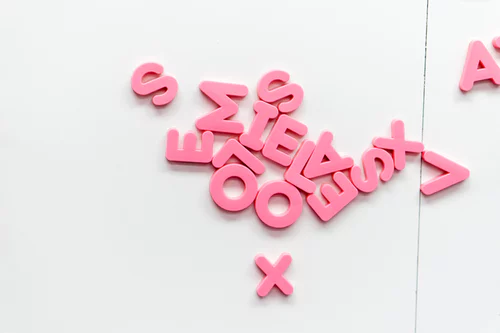# Notation and Vocabulary

## What do the letters and numbers mean?

When letters are used in maths they represent a variable. This variable is a number that may change.

• For example, the price of petrol varies, somedays petrol costs more than other days.
• Because it varies we can’t say that the price of petrol is alway one number. Therefore we represent this variable with a letter. In the case of petrol I might decide to use the letter p ( I can use ANY letter).## Algebraic vocabulary

There are several key terms that you need to learn.

Co-efficient

1. Definition: The coefficient of a term is a constant quantity which multiplies a variable. Put more simply, it’s the number that goes in front of a letter.
2. Example: __4__y = 4 is the coefficient of y. __5a__b = 5a is the coefficient of b.

Term

1. Definition: In maths the word Term describes a variable, this can be a number or number of variables multiplied together. They are separated by + and - .
2. Example: __3a __- 7y + 9ab - 2a2. The different colours represent the different Terms in the expression.

Factor

1. Definition: A factor is a number that can be divided into another number or expression without a remainder.
2. Example: 4a + 16b. 4 is a factor of 4a because 4 x a = 4a, 4a ÷ 4 = a. In this division there is no remainder. 4 is also a factor of 16b because 4 x 4b = 16b, 16b ÷ 4 = 4b. In this division there is also no remainder.

Expression

1. Definition: A collection of symbols or Terms that express a quantity when they are put together.
2. Example: 4x + 5, 5y + 9fg, 0.65t + ½ + m. These are all expressions, they are expressing a quantity of something. There is __no __equal sign!

Equation

1. Definition: An equation shows two things that are equal to each other.
2. Example: 4y + 3 = 12, 8g + 0.7y = 20 - 5t. These are equations. Equations __ALWAYS __have an equals sign ! = !!!

Identity

1. Definition: An identity is an equation that is true WHATEVER the number of the variable.
2. Example: C + C = 2C. No matter what the value of C this will __ALWAYS __be true, therefore this is an identity.
3. Counter example: 5y + 2 = 12 is __only __true when y = 2, therefore this is __not __an identity.

Formulae

1. Definition: A formulae is a mathematical equation that can be used to solve a specific problem.
2. Example: Speed = Distance/Time. The area of a triangle = ½ x height x base.

Inequality

1. Definition: An inequality describes a relationship between expressions that may be less than, less than or equal to, more than, or more than or equal to.
2. Example: 5x > 2.8t + 2 ≥ 5.
What is 5x +8c -2 in relation to the vocabulary above?
Expression
What is 46 + 2y = y + y +20 + 26?
Identity
What is 7x + 4 < 22?
Inequality